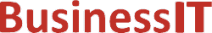O nás     Inzerce     KontaktSpolehlivé informace o IT již od roku 2011[Linux manuál]

# lgamma, lgammaf, lgammal, lgamma_r, lgammaf_r, lgammal_r: log gama funkce

Originální popis anglicky: lgamma, lgammaf, lgammal, lgamma_r, lgammaf_r, lgammal_r - log gamma function

Návod, kniha: Linux Programmer's Manual

## STRUČNĚ

```#include <math.h>

double lgamma(double x);

float lgammaf(float x);

long double lgammal(long double x);

double lgamma_r(double x, int *signp);

float lgammaf_r(float x, int *signp);

long double lgammal_r(long double x, int *signp);
```

## POPIS / INSTRUKCE

For the definition of the Gamma function, see tgamma(3).
The lgamma() function returns the natural logarithm of the absolute value of the Gamma function. The sign of the Gamma function is returned in the external integer signgam declared in <math.h>. It is 1 when the Gamma function is positive or zero, -1 when it is negative.
Since using a constant location signgam is not thread-safe, the functions lgamma_r() etc. have been introduced; they return this sign via the parameter signp.
For nonpositive integer values of x, lgamma() returns HUGE_VAL, sets errno to ERANGE and raises the zero divide exception. (Similarly, lgammaf() returns HUGE_VALF and lgammal() returns HUGE_VALL.)

## CHYBY / ERRORY

In order to check for errors, set errno to zero and call feclearexcept(FE_ALL_EXCEPT) before calling these functions. On return, if errno is non-zero or fetestexcept(FE_INVALID | FE_DIVBYZERO | FE_OVERFLOW | FE_UNDERFLOW) is non-zero, an error has occurred.
A range error occurs if x is too large. A pole error occurs if x is a negative integer or zero.

## ODPOVÍDAJÍCÍ

C99, SVID 3, BSD 4.3

## SOUVISEJÍCÍ

tgamma(3)
 2002-08-10
©2011-2020 BusinessIT.cz, ISSN 1805-0522 | Názvy použité v textech mohou být ochrannými známkami příslušných vlastníků.
Provozovatel: Bispiral, s.r.o., kontakt: BusinessIT(at)Bispiral.com | Inzerce: Best Online Media, s.r.o., zuzana@online-media.cz
O vydavateli | Pravidla webu BusinessIT.cz a ochrana soukromí | pg(9269)